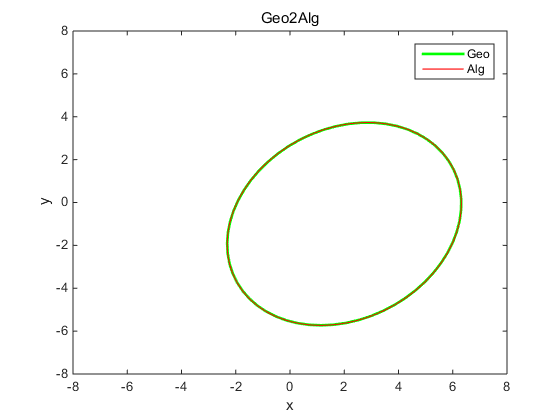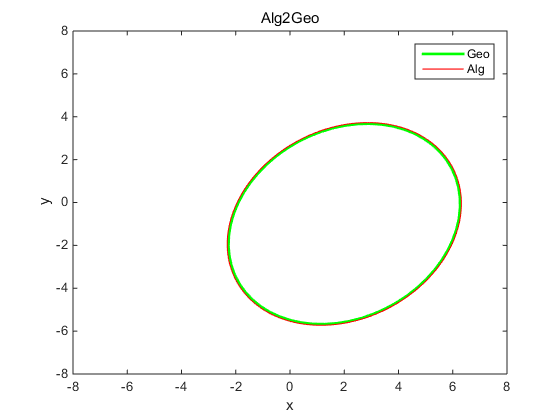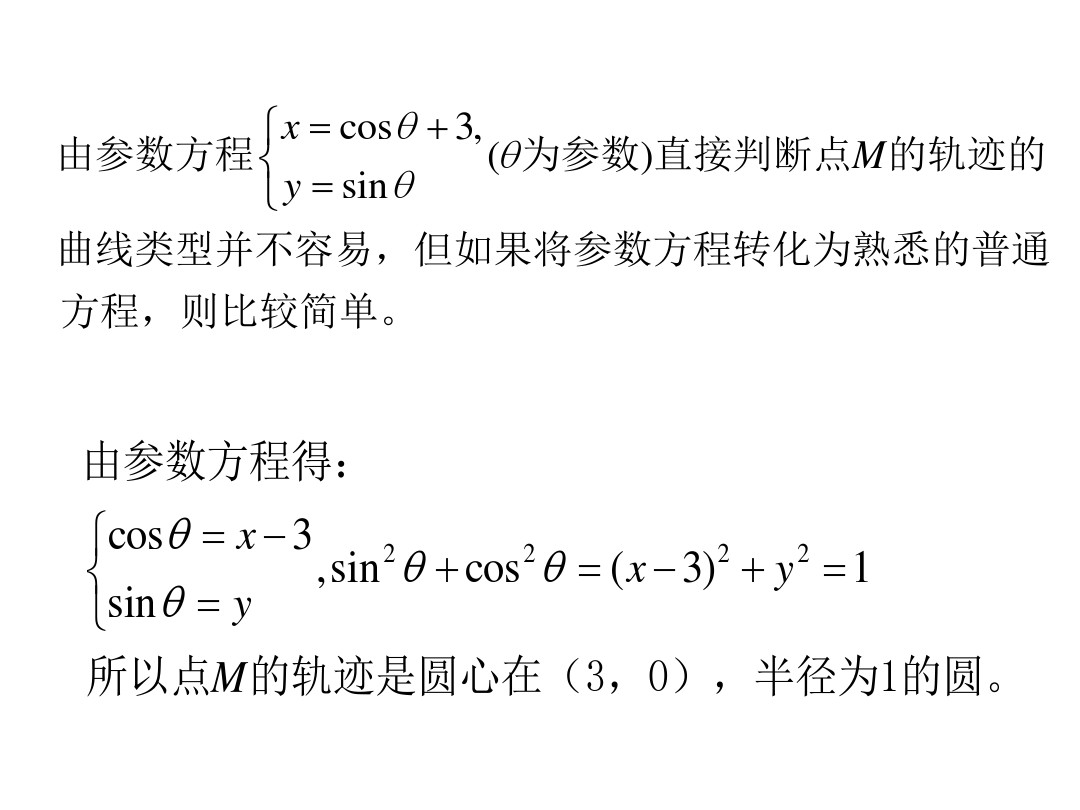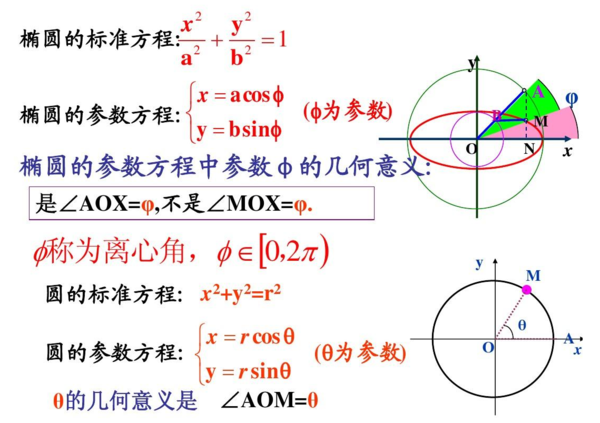• Matlab使用杂谈2-参数方程转换参数方程转换solve函数使用参数方程转换使用实例 参数方程转换 在进行数学建模时，有时候我们会得到两个具有相同变量的方程，但是如何求除这两个方程的因变量之间的关系，此时就可以...


Matlab使用杂谈2-参数方程转换
参数方程转换solve函数使用参数方程转换使用实例

参数方程转换
在进行数学建模时，有时候我们会得到两个具有相同变量的方程，但是如何求除这两个方程的因变量之间的关系，此时就可以利用参数方程转换，将参数方程转换成为y与x之间的关系式 如：y=t x=1/2t 则y=2x
solve函数使用
在进行参数方程转换前，首先引入matlab中的一个函数solve，本文只演示小部分solve函数用法，需要更复杂的用法可以点击下面有关solve函数的语法解释，具有更加详细的用法，或者点击我转载的博客 转载-Matlab中Solve函数的详细用法 Matlab中关于solve函数的语法解释 语法 S = solve(eqn,var) S = solve(eqn,var,Name,Value) Y = solve(eqns,vars) Y = solve(eqns,vars,Name,Value) [y1,…,yN] = solve(eqns,vars) [y1,…,yN] = solve(eqns,vars,Name,Value) [y1,…,yN,parameters,conditions] = solve(eqns,vars,‘ReturnConditions’,true)
参数方程转换使用实例
% 针对参数方程求解y关于x的方程
% a,b,c,d均为常量
% y=a*t+b;
% x=c*t+d;
syms a b c d t x y;
ex1=a*t+b-y==0;
ex2=c*t+d-x==0;
t=solve(ex2,t);
y=solve(subs(ex1),y)

最后可得出结果 y=b-a(d-x)/c
展开全文matlab 算法 函数式编程
• 给出了椭圆隐式方程和参数方程的互相转化，并通过MATLAB程序进行验证，实验证明了转换关系的正确性。
1. 隐式方程转参数方程
二次曲线的一般方程为：

A

x

2

+

2

B

x

y

+

C

y

2

+

2

D

x

+

2

E

y

+

F

=

0.

(1)

A{x^2}+2Bxy+C{y^2}+2Dx+2Ey+F=0. \tag{1}

若

B

2

−

A

C

<

0

B^2-AC<0

, 为椭圆；

B

2

−

A

C

=

0

B^2-AC=0

, 为抛物线；

B

2

−

A

C

>

0

B^2-AC>0

,为双曲线。 二次曲线可通过旋转和平移来变成标准方程，从而得到其几何参数。旋转的作用是消去交叉项，平移的作用是使中心为原点，下面以椭圆为例。 方程的二次项为：

[

x

,

y

]

[

A

B

B

C

]

[

x

y

]

.

(2)

[x, y] \left[ \begin{matrix} A &B\\ B &C \end{matrix} \right] \left[ \begin{matrix} x\\ y \end{matrix} \right]. \tag{2}

一次项为

2

[

x

,

y

]

[

D

E

]

.

2[x, y] \left[ \begin{matrix} D\\ E \end{matrix} \right].

我们对二次项进行旋转，消去交叉项，旋转角

θ

\theta

就是椭圆的偏角。假设新的坐标为

(

x

′

,

y

′

)

(x^{'}, y^{'})

, 那么

[

x

′

y

′

]

=

[

cos

⁡

θ

sin

⁡

θ

−

sin

⁡

θ

cos

⁡

θ

]

[

x

y

]

.

\left[ \begin{matrix} x^{'}\\ y^{'}\\ \end{matrix} \right]= \left[ \begin{matrix} \cos\theta & \sin\theta\\ -\sin\theta &\cos\theta\\ \end{matrix} \right] \left[ \begin{matrix} x\\ y\\ \end{matrix} \right].

将

[

x

y

]

=

[

cos

⁡

θ

−

sin

⁡

θ

sin

⁡

θ

cos

⁡

θ

]

[

x

′

y

′

]

\left[ \begin{matrix} x \\ y \\ \end{matrix} \right]= \left[ \begin{matrix} \cos\theta &-\sin\theta\\ \sin\theta & \cos\theta\\ \end{matrix} \right]\left[ \begin{matrix} x^{'}\\ y^{'}\\ \end{matrix} \right]

带入式(2)，然后令矩阵(1,2) 位置的元素为0，可得，

(

C

−

A

)

sin

⁡

2

θ

+

2

B

cos

⁡

2

θ

=

0

,

(C-A)\sin2\theta+2B\cos2\theta=0,

那么，

tan

⁡

2

θ

=

2

B

A

−

C

.

\tan2\theta=\frac{2B}{A-C}.

那么原来的二次项矩阵变为：

[

cos

⁡

θ

sin

⁡

θ

−

sin

⁡

θ

cos

⁡

θ

]

[

A

B

B

C

]

[

cos

⁡

θ

−

sin

⁡

θ

sin

⁡

θ

cos

⁡

θ

]

=

[

A

′

0

0

C

′

]

.

\left[ \begin{matrix} \cos\theta &\sin\theta\\ -\sin\theta &\cos\theta\\ \end{matrix} \right] \left[ \begin{matrix} A & B\\ B& C\\ \end{matrix} \right] \left[ \begin{matrix} \cos\theta &-\sin\theta\\ \sin\theta & \cos\theta \end{matrix} \right]= \left[ \begin{matrix} A^{'} & 0\\ 0& C^{'}\\ \end{matrix} \right].

两边同时乘以旋转矩阵的逆，并比较两边矩阵的(1,1)和(2,2)位置元素可得：

A

′

=

A

+

B

tan

⁡

θ

,

C

′

=

C

−

B

tan

⁡

θ

.

A^{'}=A+B\tan\theta, C^{'}=C-B\tan\theta.

对于一次项，做同样的旋转操作可得，

[

D

′

E

′

]

=

[

cos

⁡

θ

sin

⁡

θ

−

sin

⁡

θ

cos

⁡

θ

]

[

D

E

]

=

[

D

cos

⁡

θ

+

E

sin

⁡

θ

−

D

sin

⁡

θ

+

E

cos

⁡

θ

]

.

\left[ \begin{matrix} D^{'}\\ E^{'} \end{matrix} \right]= \left[ \begin{matrix} \cos\theta & \sin\theta\\ -\sin\theta & \cos\theta\\ \end{matrix} \right] \left[ \begin{matrix} D\\ E\\ \end{matrix} \right]= \left[ \begin{matrix} D\cos\theta+E\sin\theta\\ -D\sin\theta+E\cos\theta\\ \end{matrix} \right].

那么化简后的椭圆方程为

A

′

x

′

2

+

C

′

y

′

2

+

2

D

′

x

′

+

2

E

′

y

′

+

F

=

0.

A^{'}x^{'}{^2}+C^{'}y^{'}{^2}+2D^{'}x^{'}+2E^{'}y^{'}+F=0.

我们对它进一步配方化为标准椭圆方程：

(

x

′

+

D

′

/

A

′

)

2

D

′

2

A

′

2

+

E

′

2

C

′

A

′

−

F

A

′

+

(

y

′

+

E

′

/

C

′

)

2

D

′

2

A

′

C

′

+

E

′

2

C

′

2

−

F

C

′

=

1

,

\frac{(x^{'}+D^{'}/A^{'})^2}{\frac{D^{'}{^2}}{A^{'}{^2}}+\frac{E^{'}{^2}}{C^{'}A^{'}}-\frac{F}{A^{'}}}+\frac{(y^{'}+E^{'}/C^{'})^2}{\frac{D^{'}{^2}}{A^{'}C^{'}}+\frac{E^{'}{^2}}{C^{'}{^2}}-\frac{F}{C^{'}}}=1,

化简后我们得到椭圆5个参数

(

x

c

,

y

c

,

a

,

b

,

θ

)

(x_c, y_c, a,b,\theta)

为

{

x

c

=

B

E

−

C

D

A

C

−

B

2

y

c

=

B

D

−

A

E

A

C

−

B

2

a

=

x

c

2

+

(

y

c

2

(

C

−

B

tan

⁡

θ

)

−

F

)

A

+

B

tan

⁡

θ

b

=

y

c

2

+

(

x

c

2

(

A

+

B

tan

⁡

θ

)

−

F

)

C

−

B

tan

⁡

θ

tan

⁡

2

θ

=

2

B

A

−

C

(

θ

=

1

2

arctan

⁡

2

B

A

−

C

)

.

\left\{\color{blue}{ \begin{array}{rcl} &x_c=\frac{BE-CD}{AC-B^2} \\ &y_c=\frac{BD-AE}{AC-B^2}\\ &a=\sqrt{\frac{x_c^2+(y_c^2(C-B\tan\theta)-F)}{A+B\tan\theta}}\\ &b=\sqrt{\frac{y_c^2+(x_c^2(A+B\tan\theta)-F)}{C-B\tan\theta}}\\ &\tan2\theta=\frac{2B}{A-C}(\theta=\frac{1}{2}\arctan{\frac{2B}{A-C}}) \end{array}}. \right.

将相应的值带入就好。

注

意

二

次

型

(

式

1

)

系

数

是

否

有

2

\color{red}{注意二次型 (式1) 系数是否有2}

.
2. 参数方程化为隐式方程
假设椭圆5参数

(

x

c

,

y

c

,

a

,

b

,

θ

)

(x_c, y_c, a,b,\theta)

, 其中

a

≥

b

a\geq b

。它对应的标准方程为

x

2

a

2

+

y

2

b

2

=

1.

\frac{x^2}{a^2}+\frac{y^2}{b^2}=1.

通过坐标变换（旋转+平移）把标准坐标变到一般坐标：

[

x

′

y

′

]

=

[

cos

⁡

θ

sin

⁡

θ

−

sin

⁡

θ

cos

⁡

θ

]

[

x

y

]

+

[

x

c

y

c

]

.

\left[ \begin{matrix} x^{'}\\ y^{'}\\ \end{matrix} \right]= \left[ \begin{matrix} \cos\theta & \sin\theta\\ -\sin\theta &\cos\theta\\ \end{matrix} \right] \left[ \begin{matrix} x\\ y\\ \end{matrix} \right]+\left[ \begin{matrix} x_c\\ y_c\\ \end{matrix} \right].

反解出

(

x

,

y

)

(x, y)

带入标准方程，通过简单的化简可得：

{

A

=

cos

⁡

2

θ

a

2

+

sin

⁡

2

θ

b

2

B

=

sin

⁡

θ

cos

⁡

θ

(

1

a

2

−

1

b

2

)

C

=

sin

⁡

2

θ

a

2

+

cos

⁡

2

θ

b

2

D

=

−

x

c

cos

⁡

2

θ

−

y

c

sin

⁡

θ

cos

⁡

θ

a

2

+

−

x

c

sin

⁡

2

θ

+

y

c

sin

⁡

θ

cos

⁡

θ

b

2

E

=

−

y

c

sin

⁡

2

θ

−

x

c

sin

⁡

θ

cos

⁡

θ

a

2

+

−

y

c

cos

⁡

2

θ

+

x

c

sin

⁡

θ

cos

⁡

θ

b

2

F

=

(

x

c

cos

⁡

θ

+

y

c

sin

⁡

θ

)

2

a

2

+

(

x

c

sin

⁡

θ

−

y

c

cos

⁡

θ

)

2

b

2

−

1

.

\left\{\color{blue}{ \begin{aligned} &A=\frac{\cos^2\theta}{a^2}+\frac{\sin^2\theta}{b^2} \\ &B=\sin\theta\cos\theta(\frac{1}{a^2}-\frac{1}{b^2})\\ &C=\frac{\sin^2\theta}{a^2}+\frac{\cos^2\theta}{b^2} \\ &D=\frac{-x_c\cos^2\theta-y_c\sin\theta\cos\theta}{a^2}+\frac{-x_c\sin^2\theta+y_c\sin\theta\cos\theta}{b^2}\\ &E=\frac{-y_c\sin^2\theta-x_c\sin\theta\cos\theta}{a^2}+\frac{-y_c\cos^2\theta+x_c\sin\theta\cos\theta}{b^2} \\ &F=\frac{(x_c\cos\theta+y_c\sin\theta)^2}{a^2}+\frac{(x_c\sin\theta-y_c\cos\theta)^2}{b^2}-1&\\ \end{aligned}}. \right.

注

意

二

次

型

(

式

1

)

系

数

是

否

有

2

\color{red}{注意二次型 (式1) 系数是否有2}

.
3.MATLAB代码验证
%%=====================
%椭圆参数方程和隐式方程互化
%input: geometric parameter [xc,yc,a,b,theta], suppose a>=b;
%       or algebraic parameter [A,B,C,D,E,F];
%
%output: the transformed result
%%=======================
function [result]=geo2alg(parameter)
close all;
if (nargin==0)
%parameter=[2,-1,5,4,1]; % geometric parameter
parameter=[0.0559   -0.0102    0.0466   -0.1221    0.0670   -0.6888];% algeraic
end
%-----------Geometric to Algebraic-----------%
if(size(parameter,2)==5)

%geometric
xc=parameter(1);
yc=parameter(2);
a=parameter(3);
b=parameter(4);
theta=parameter(5);

% convert to algebraic coefficients
A=cos(theta)^2/a^2+sin(theta)^2/b^2;
B=sin(theta)*cos(theta)*(1/(a^2)-1/(b^2));
C=sin(theta)^2/a^2+cos(theta)^2/b^2;
D=(-xc*cos(theta)^2-yc*sin(theta)*cos(theta))/a^2+(-xc*sin(theta)^2+yc*sin(theta)*cos(theta))/b^2;
E=(-yc*sin(theta)^2-xc*sin(theta)*cos(theta))/a^2+(-yc*cos(theta)^2+xc*sin(theta)*cos(theta))/b^2;
F=(xc*cos(theta)+yc*sin(theta))^2/a^2+(xc*sin(theta)-yc*cos(theta))^2/b^2-1;

result=[A,B,C,D,E,F];
end
%-----------Algebraic to Geometric-----------%
if(size(parameter,2)==6)

%algebraic
A=parameter(1);
B=parameter(2);
C=parameter(3);
D=parameter(4);
E=parameter(5);
F=parameter(6);

% convert to geometric
theta=1/2*atan2(2*B,(A-C));
xc=(B*E-C*D)/(A*C-B^2);
yc=(B*D-A*E)/(A*C-B^2);
a=sqrt(xc^2+(yc^2*(C-B*tan(theta))-F)/(A+B*tan(theta)));
b=sqrt(yc^2+(xc^2*(A+B*tan(theta))-F)/(C-B*tan(theta)));

result=[xc,yc,a,b,theta];
end
%-------------Plot Two Modes-------------%
%geometric
t= linspace(0,2*pi,60);
x=a*cos(t);
y=b*sin(t);
Ex = x*cos(theta)-y*sin(theta) + xc;
Ey = x*sin(theta)+y*cos(theta) + yc;
figure
plot(Ex,Ey,'g-','linewidth',2);
hold on;
%algebraic
syms x y;
z1=ezplot(A*x^2+2*B*x*y+C*y^2+2*D*x+2*E*y+F,[-8,8]);
set(z1,'Color','r');
legend('Geo','Alg');
if (size(parameter,2)==5)
title('Geo2Alg');
else
title('Alg2Geo');
end
end

结果： 1.Geo2Alg input: parameter=[2,-1,5,4,1]; output: result=[0.0559 -0.0102 0.0466 -0.1221 0.0670 -0.6888]; 几何参数转代数系数，绿色是几何作图，红色为转换的代数作图，两图重合.2.Alg2Geo input: parameter=[0.0559 -0.0102 0.0466 -0.1221 0.0670 -0.6888]; output: result=[2.0019 -0.9996 3.9593 4.9450 -0.5715]; 代数系数转几何参数，绿色为转换的几何作图，红色为代数系数作图，两图重合。展开全文线性代数 矩阵
• 一、曲线的参数方程 1.1 参数方程的概念 一般地，在平面直角坐标系中，如果曲线上任意一点的坐标x,yx,yx,y都是某个变数ttt的函数 {x=f(t)y=g(t)(1) \left\{ \begin{aligned} &x=f(t)\\ &y=g(t) \end{aligned...
一、曲线的参数方程
1.1 参数方程的概念
一般地，在平面直角坐标系中，如果曲线上任意一点的坐标

x

,

y

x,y

都是某个变数

t

t

的函数

{

x

=

f

(

t

)

y

=

g

(

t

)

(1)

\left\{ \begin{aligned} &x=f(t)\\ &y=g(t) \end{aligned} \right.\tag{1}

并且对于每个

t

t

的允许值，由方程组（1）所确定的点

M

(

x

,

y

)

M(x,y)

都在这条曲线上，那么方程组（1）就称为这条曲线的参数方程，联系变数

x

,

y

x,y

的变数

t

t

叫做参变数，简称参数。相对参数方程而言，直接给出点的坐标间关系的方程叫做普通方程。这里的参数

t

t

可以是一个有物理意义或几何意义的变数，也可以是没有明显实际意义的变数。
一个曲线多个参数方程，一个参数方程却只能对应一条曲线；此外，在建立参数方程时应该注明参数和参数的取值范围。
1.2 圆的参数方程
圆心在原点，半径为

r

r

,

θ

\theta

为转过的角度。

{

x

=

r

cos

⁡

θ

y

=

r

sin

⁡

θ

θ

∈

[

0

,

2

π

)

(2)

\left\{ \begin{aligned} & x=r\cos\theta\\ & y=r\sin\theta \end{aligned}\quad \theta\in[0,2\pi) \right.\tag{2}

化成普通方程便于观察曲线的类型。另外，若圆心不在原点，若圆心为

(

a

,

b

)

(a,b)

，则对应参数方程应该为：

{

x

=

a

+

r

cos

⁡

θ

y

=

b

+

r

sin

⁡

θ

θ

∈

[

0

,

2

π

)

(3)

\left\{ \begin{aligned} & x=a+r\cos\theta\\ & y=b+r\sin\theta \end{aligned}\quad \theta\in[0,2\pi) \right.\tag{3}

1.3 参数方程和普通方程的互化
一般地可以通过消去参数从而将参数方程转化成普通方程；若已知普通方程，可以通过令

x

=

f

(

t

)

x=f(t)

，再将

x

x

带入普通方程来转化成参数方程。特别注意

x

,

y

,

θ

x,y,\theta

的取值范围。
二、圆锥曲线
2.1 椭圆的参数方程
长轴长为a，短轴长为b的椭圆的普通方程为：

x

2

a

2

+

y

2

b

2

=

1

（

a

>

b

>

0

）

(4)

对应的参数方程为：

{

x

=

a

cos

⁡

ϕ

y

=

b

sin

⁡

ϕ

ϕ

∈

[

0

,

2

π

)

(5)

\left\{ \begin{aligned} &x=a\cos\phi\\ &y=b\sin\phi\\ \end{aligned} \right. \quad \phi\in[0,2\pi)\tag{5}

2.2 双曲线的参数方程
若双曲线的普通方程为：

x

2

a

2

−

y

2

b

2

=

1

（

a

>

0

b

>

0

）

(5)

则对应的参数方程为：

{

x

=

a

sec

⁡

ϕ

y

=

b

sin

⁡

ϕ

ϕ

∈

[

0

,

2

π

)

(6)

\left\{ \begin{aligned} &x=a\sec\phi\\ &y=b\sin\phi\\ \end{aligned} \right. \quad \phi\in[0,2\pi)\tag{6}

ϕ

\phi

为参数，满足条件：

ϕ

∈

[

0

,

2

π

)

\phi\in[0,2\pi)

ϕ

≠

π

2

ϕ

≠

3

π

2

python中似乎没有

sec

⁡

\sec

函数，总之等于余弦的倒数就对了：

sec

⁡

ϕ

=

1

c

o

s

ϕ

\sec\phi=\frac{1}{cos\phi}

2.3 抛物线方程
设抛物线普通方程为

y

2

=

2

p

x

(7)

y^2=2px\tag{7}

其中，

p

p

表示焦点在顶点的距离。参数方程如下：

{

x

=

2

p

tan

⁡

2

α

y

=

2

p

tan

⁡

α

(8)

\left\{ \begin{aligned} &x=\frac{2p}{\tan^2\alpha}\\ &y=\frac{2p}{\tan\alpha} \end{aligned}\tag{8} \right.

令

t

=

1

tan

⁡

α

t=\frac{1}{\tan\alpha}

，(8)变成：

{

x

=

2

p

t

2

y

=

2

p

t

(9)

\left\{ \begin{aligned} &x=2pt^2\\ &y=2pt \end{aligned}\tag{9} \right.

t

∈

(

−

∞

,

0

)

∪

(

0

,

+

∞

)

t\in(-\infty,0)\cup(0,+\infty)

，

α

\alpha

除顶点外任意一点与顶点连线与

O

x

Ox

夹角，显然当

t

=

0

t=0

时恰好是抛物线原点，

t

t

表示除原点外任意一点与原点连线斜率的倒数。
三、直线
过点

P

0

(

x

0

,

y

0

)

P_0(x_0,y_0)

倾角为

α

\alpha

的直线

l

l

，其普通方程为：

y

−

y

0

=

tan

⁡

α

(

x

−

x

0

)

(10)

y-y_0=\tan\alpha(x-x_0)\tag{10}

对应的参数方程为：

{

x

=

x

0

+

t

cos

⁡

α

y

=

y

0

+

t

sin

⁡

α

(11)

\left\{ \begin{aligned} &x=x_0+t\cos\alpha\\ &y=y_0+t\sin\alpha \end{aligned} \right.\tag{11}

t

t

为参数，其绝对值等于动点

P

P

到

P

0

P0

的距离，即：

∣

t

∣

=

∣

P

P

0

∣

(12)

|t|=|PP0|\tag{12}

四、渐开线和摆线
4.1 渐开线参数方程，
渐伸线（involute）(或称渐开线(evolvent))和渐屈线（evolute）是曲线的微分几何上互为表里的概念。若曲线A是曲线B的渐伸线，曲线B是曲线A的渐屈线。在曲线上选一定点

S

S

。有一动点P由S出发沿曲线移动，选在

P

P

的切线上的

Q

Q

，使得曲线长

S

P

SP

和直线段长

P

Q

PQ

相同。渐伸线就是

Q

Q

的轨迹。圆的渐开线方程为：

{

x

=

r

(

cos

⁡

ϕ

+

ϕ

sin

⁡

ϕ

)

y

=

r

(

sin

⁡

ϕ

−

ϕ

cos

⁡

ϕ

)

(13)

\left\{ \begin{aligned} &x=r(\cos\phi+\phi\sin\phi)\\ &y=r(\sin\phi-\phi\cos\phi) \end{aligned} \right.\tag{13}

ϕ

\phi

是参数。
在机械工业中，广泛地使用齿轮传递动力。由于渐开线的齿形的齿轮磨损少、传动平稳，制造安装较为方便。因此大多数齿轮采用这种齿形，设计加工这种齿轮，需要借助圆的渐开线方程。
4.2 摆线对应的参数方程为：

{

x

=

r

(

ϕ

−

sin

⁡

ϕ

)

y

=

r

(

1

−

cos

⁡

ϕ

)

(14)

\left\{ \begin{aligned} &x=r(\phi-\sin\phi)\\ &y=r(1-\cos\phi) \end{aligned} \right.\tag{14}

ϕ

\phi

是参数。
展开全文• 参数方程中参数的意义： 参数方程中t的几何意义要看具体的曲线方程了，一般都是长度，角度等几何量，也有一些是不容易找到对应的几何量的。 参数方程定义： 一般的，在平面直角坐标系中，如果曲线上任意一点的...
目录
参数方程中参数的意义：
参数方程定义：
什么是参数方程：
参数方程与普通方程的公式：
举例：
参数方程：

参数方程中参数的意义：
参数方程中t的几何意义要看具体的曲线方程了，一般都是长度，角度等几何量，也有一些是不容易找到对应的几何量的。

参数方程定义：
一般的，在平面直角坐标系中，如果曲线上任意一点的坐标x，y都是某个变数t的函数{x=f(t)，y=g(t)并且对于t的每一个允许值，由上述方程组所确定的点M（x,y)都在这条曲线上，那么上述方程则为这条曲线的参数方程，联系x，y的变数t叫做变参数，简称参数，相对于参数方程而言，直接给出点的坐标间关系的方程叫做普通方程。

什么是参数方程：
其实就是 ：
y=f（t）；x=g（t）；其中t是参数，分别能表示出x，y；你看看下面参数方程与一般函数的转化你就明白了；

参数方程与普通方程的公式：
参数方程与普通方程的互化最基本的有以下四个公式：
1.cos²θ+sin²θ=1
2.ρ=x²+y²
3.ρcosθ=x
4.ρsinθ=y
举例：
参数方程：
一般的参数方程，主要使2式子进行乘除运算消掉  t。
遇到三角三角函数一般使用公式带入，消掉。
x=3-2t ①  y=-1－4t ②
解： ①×2-②得 x-2y=2(3-2t)-(-1-4t) x-2y=7 ∴2x-y = 7

将x， y的中参数转化为同一的，之后进行替换，得出一般函数方程。
例子：
x=cosθ （θ为参数） ① y=cos2θ+1 ② 由②得 y=2cos²θ-1+1 y=2cos²θ 由①得 cosθ=x ∴y=2x² -1

例：又例圆，椭圆等：展开全文• 设主动变换方程，即把直椭圆逆时针旋转的表达式为： 或者： 代入直椭圆表达式，得： 然后待定系数，解方程以下就可以了。 下面李阳 的答案是线性代数中对角化二次型的方法，也不错： 即寻找...
• 一、圆和椭圆的参数方程的由来 从下面的动画中能总结出来圆和椭圆的参数方程吗？ 圆的参数方程（圆心$$(a，b)$$，半径$$R$$） $$\begin{cases} x=a+Rcos\theta \\ y=b+Rsin\theta\end{cases}(\theta为参数，0\leq ... • ## 高数——隐函数与参数方程求导 千次阅读 多人点赞 2019-10-18 11:19:16 如果方程f(x,y)=0能确定y是x的函数，那么称这种方式表示的函数是隐函数。而函数就是指：在某一变化过程中，两个变量x、y，对于某一范围内的x的每一个值，y都有确定的值和它对应，y就是x的函数。这种关系一般用y=f(x)... • 线性方程的几何意义二元线性方程 该方程是一个二元线性方程组，包含两个方程，每个方程是一条直线，两条直线的交点就是该方程有唯一解，这就是二元线性方程的几何意义。平面方程 空间内不在同一直线上的三点构成一...直线 向量 • 文章目录空间直线的参数方程空间直线的向量式方程直线的点向式方程空间直线的两点式方程空间直线的一般方程空间直线的一般方程化为点向式方程小结：直线方程的几种形式参考资料 直线是随处可见的空间形状 问题:如何... • 高考主要考查直线和圆的极坐标方程，参数方程与普通方程的互化，常见曲线的参数方程参数方程的简单应用，以极坐标、参数方程与普通方程的互化为主要考查形式，同时考查直线与曲线位置关系等解析几何知识.... • 如果方程中的参数是符号参数，则只返回 M、K 和 I。 对于数值解的情况，还返回 A 和 B。 对于较小的系统（1 到 10 个左右的状态），如果需要，用户通常可以计算符号 A 和 B。 对于较大的系统，符号 A 和 B 矩阵通常...matlab • 如直线\(\left\{\begin{array}{l}{x=1+t①}\\{y=2-t②}\end{array}\right.(t为参数)$$， 将$$t=x-1$$代入②，得到$$y=2-(x-1)$$， 即$$x+y-3=0$$，代入消参完成。 ㊁加减消参法 依上例，两式相加，得到\...
• 一、常用参数方程我们需要掌握的参数方程，和我们学习过的一些曲线方程相关，与直角坐标方程的互换等，这些需要掌握，首先我们开始复习一下参数方程的基础知识，在这里不做过多的讲解，主要是回忆巩固一下。...
• 线性方程组的求法与示例详解 线性方程组 由n个1维未知量，m个方程组成的组合叫做线性方程组。 特别的当方程组右边的值全都是0时叫做齐次线性方程组。 增广矩阵 在系数矩阵的右边添上一列，该列由线性方程组...线性代数 矩阵
• 通过对 BWRS 方程中各个参数的分析研究，为用 BWRS 状态方程求解天然气物性参数的单位制变换提供了有力证据， 给出了详细的分析过程； 通过用该方法求解天然气的热物性参数的计算实例，得出了利用类似方法进行单位制...
• 假设悬索桥主缆自重沿弧长均匀分布，加劲梁、桥面等其余恒载沿水平均匀分布，导出了悬索桥主缆成桥线形的参数方程解。然后由边界条件及连续性条件，建立了确定主缆成桥线形的非线性方程组。根据中跨方程组可求出成桥...
• 例7 已知椭圆的参数方程为 x=acost y=bsint 求椭圆在t=π/4相应点的切线方程。 解： 想找切线方程，就是要求导数。 参数方程的导数根据公式，①求dy/dt ②求dx/dt ③两者相除 clear syms x y a b t fy=b*sin(t...
• 希望把如下曲线的参数方程变成隐函数F(x,y)=0F(x,y)=0形式: {x=y=−9sin(2t)−5sin(3t)9cos(2t)−5cos(3t)\left\{\;\; \begin{array}{rl} x=&-9 \sin (2 t)-5 \sin (3 t) \\ y=&9 \cos (2 t)-5 \cos (3 t) \\ \...符号计算 隐函数 Macaulay2
• 一个在XOY中的标准的椭圆 X^2/A^2 + Y^2/B^2 = 1 在UOV中满足的方程就变成了 [U*COS(θ) - V*SIN(θ)]^2/A^2 +[U*SIN(θ) + V*COS(θ)]/B^2 = 1 U^2{[BCOS(θ)]^2 +[ASIN(θ)]^2} +V^2{[BSIN(θ)]^2 +[ACOS(θ)]^2...
• 解疑06 如何把一个直线的参数方程的一般式转化为标准式？ 答：我们注意到 $$\begin{cases} x=x_0+cos\theta\cdot t \\ y=y_0+sin\theta\cdot t \end{cases}(t为参数)$$ 方程组中的参数 $$t$$ 的两个系数的平方和是...
• matlab求参数方程二次导函数代码 开发人员手册 涵盖各种计算机体系结构，编程语言，安全性，网络，游戏开发，图形编程，敏捷开发等的开发人员手册。该手册还包括应用程序，框架，库和工具，它们将使您变得更好，更...
• 这是我所要用的二阶微分方程，现已通过试验得到数据，想通过该方程拟合数据得到方程中的四个参数，通过查询有说二阶微分方程转化为差分方程或者积分方程，然后MATLAB编程拟合，想知道如何转化为差分方程或者积分方程...c++ c语言
• 先把标题放在这里,内容我慢慢准备,一点点添加1. 一个简单的例子从最简单的情况出发,连续可微的封闭曲线为边界的,最简单例子是”圆”....所以我选择了如下参数方程的椭圆： {x=y=5costcosθ−3sints微分几何 计算几何 算法
• 关于matlab读取大量文件及MATLAB处理变参数多元非线性方程组 循环读取大量文件 前段时间用MATLAB处理txt文件时遇到了一个问题，由于txt文件太多因此需要做成循环来依次处理500（实际上有5000多个）多个txt文件。最...matlab
• ## 将方程转换为矩阵

千次阅读 2020-06-25 20:49:26
方程转换为矩阵，我只想到矩阵的加法： 0.25∗[210414161534852t]+[−40−40−40−40] 0.25* \left[ \begin{matrix} 2104\\ 1416\\ 1534\\ 852t \end{matrix} \right] + \left[ \begin{matrix} -40\\ -...
• B、借助直线的参数方程的参数$$t$$的几何意义考察，比如求线段的长度； C、借助平面几何知识考察，比如求倾斜角等； D、借助极坐标考查面积，线段长度等， E、借助解析几何考查，比如相关点法求轨迹， F、借助...
• 然后，采用低通滤波器从实测电磁功率中获取缓变功率曲线，从而将含有不可测变量的运动方程转化为以待辨识参数为未知量的可辨识方程。应用结果表明所提方法能够减少参数辨识中不考虑机械功率变化或者认为机械功率变化......